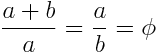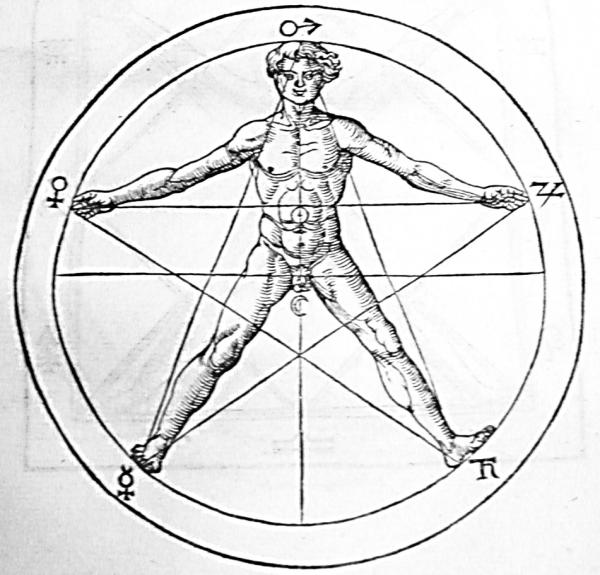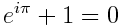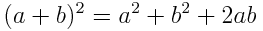# Mathematics and Beauty

Friday, September 22, 2017

Mathematics when rightly viewed, possesses not only truth but supreme beauty. — Bertrand Russel

What has Mathematics to do with the beauty and does it even matter? Yes, it does. For some of us, the enjoyment of beauty of mathematics is what encourages to study the subject.

A mathematician can find beauty in methods (such as proofs) or in results or in theorems or even in numbers.

Proofs are to Mathematics what spelling is to poetry. — Vladimir Arnold

Take for example, the proof that Zero is an even number. In other words, its parity—the quality of an integer being even or odd—is even.

The simplest way to prove that zero is even is to check if it fits the definition of even: Is zero divisible by 2? Yes, it is an integer multiple of 2, specifically 0 × 2. As a result, zero shares all the properties that characterize even numbers: 0 is neighbored on both sides by odd numbers, 0 is the sum of an integer (0) with itself, and a set of 0 objects can be split into two equal sets. The parity rules of arithmetic, such as even − even = even, require 0 to be even. Thus, zero is an even number.
Not only is 0 divisible by 2, it is the only number that is divisible by every power of 2, which makes 0 the most even number of all.

Now, let’s suppose for a moment that zero is not an even number i.e. zero is an odd number. It means, it must satisfy the definition of odd, hence, zero can be represented in the form of 2k + 1 where k ∈ Z (integers). This means, 0 = 2k + 1 ⇒ k = -1/2 i.e. k is a rational number (not integer). This contradicts our assumption. Hence, zero is not an odd number i.e. zero is an even number.

Now, I’ve proved zero is an even number and also (of course) zero is not an odd number. So, isn’t it fascinating how easily one statement, in mathematics, can be proved in different ways.
The theorem for which the greatest number of different proofs have been discovered is possibly the Pythagorean theorem1, with hundreds of proofs having been published.

Now, let’s talk about the mathematicians that find beauty in objects, shapes. In mathematics, two quantities are in the golden ratio if their ratio is the same as the ratio of their sum to the larger of the two quantities. For quantities a and b such that a, b > 0:It comes out to be an irrational number (φ = 1.61805…), called the golden ratio2.

Some of the great works of the 20th century are based on the golden rectangle, a rectangle whose side lengths are in the golden ratio – believing this proportion to be aesthetically pleasing. such as Leonardo da Vinci’s Vitruvian Man3, showing the golden ratio in body dimensions.Some mathematicians see beauty in mathematical results that establish connections between two areas of mathematics that at first sight appear to be unrelated. For example, Euler’s identity:which relates five mathematical constants in a concise and elegant way.

Now, the question arises: Why should the non-mathematician care about things of this nature?
The foremost reason is that mathematics is beautiful, even if it is, sadly, more inaccessible than other forms of art. The second is that it is useful. Mathematics is founded on simple yet powerful elements — numbers. Numbers, just like the elements of nature, share a complex relationship with every other element in that set. This relationship is universally valid.
The equation:stands true on Earth, on Jupiter and even if the galaxies collide. Because, mathematics doesn’t exist in the physical dimension. It exists entirely in the human mind, it’s a mental concept, and I think this is the most beautiful concept ever made by the human mind. Mathematics and human beings are co-dependent to aid each other’s growth.
And, this is the reason:

If beauty is truth, and truth is beauty, then surely mathematics is the most beautiful thing of all.

Footnotes:
1: Pythagorean theorem:
2: Golden ratio:
3: Vitruvian Man: